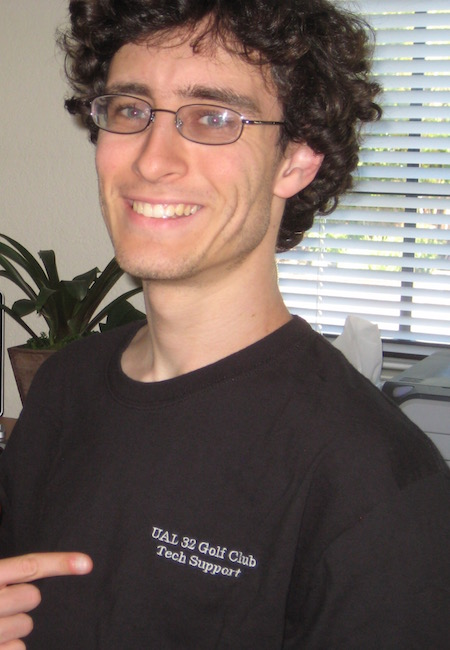Contact
cdoneill «at» sdsu.edu
GMCS Building, Room 570
Math & Stats Department
San Diego State University
5500 Campanile Dr
San Diego, CA 92182
U.S.A.
What is a Numerical Semigroup?
Numerical semigroups stem from the classic problem of making exact change. The United States uses coins worth 1, 5, 10, and 25 cents, so there are 18 ways make change for 33 cents (for instance, one could use 1 quarter and 8 pennies, or simply 33 pennies; it is a fun and enlightening exercise to find them all!). On the other hand, the European Union uses coins worth 1, 2, 10, and 20 euro cents, so there are a whopping 47 ways to make change for 33 euro cents:
• 17 ways using only 1- and 2-cent coins;
• 12 ways using a single 10-cent coin and no 20-cent coins;
• 7 ways using 2 10-cent coins (and no 20-cent coins);
• 2 ways using 3 10-cent coins; and
• 9 ways using a single 20-cent coin.
Finding all possible combinations can be tricky, but exact change can always be made in some way for any (non-negative integer) amount since both of these coin systems have a coin worth 1 cent.
But what if a country had only had 2 coins, worth 3 and 8 cents, respectively? Now, there are some values for which change cannot be made! It turns out 13 is the largest such value; it is not hard to check that one cannot make exactly 13 cents using coins worth 3 and 8 cents, but why is it the largest? Well, 14 cents can be made using one 8-cent coin and 2 3-cent coins, 15 cents can be made with 5 3-cent coins, and 16 can be made with 2 8-cent coins, at which point any larger amount can be obtained from one of these 3 by including an appropriate number of 3-cent coins.
More formally, a numerical semigroup $$S$$ with generators $$n_1, \ldots, n_k$$ is the set $S = \langle n_1, \ldots, n_k \rangle = \{a_1n_1 + \cdots + a_kn_k : a_1, \ldots, a_k \in \mathbb Z_{\ge 0}\}$ consisting of all non-negative integer combinations of $$n_1, \ldots, n_k$$. For instance, the elements of $$\langle 3, 8 \rangle$$ are the integers for which change can be made using coins worth 3 and 8 cents, respectively. As hinted above, one classic question is as follows: what is the largest integer lying outside of a given numerical semigroup $$S$$? Thinking of the values $$n_1, \ldots, n_k$$ as coin denominations, this is asking: what is the largest value for which we cannot make exact change? This value is known as the Frobenius number of $$S$$, and is often denoted $$\mathsf F(S)$$. So long as the generators $$n_1, \ldots, n_k$$ do not all have some common factor (that is, if $$\gcd(n_1, \ldots, n_k) = 1$$), there will indeed be a largest such integer, but finding it in terms of $$n_1, \ldots, n_k$$ is known to be a difficult computational problem in general.
From these humble beginnings, numerical semigroups have become ubiquitous objects appearing across the mathematical spectrum. They are often studied using tools from algebra, combintorics, number theory, and more recently polyhedral geometry, but have also worked their way into a host of applications, including algebraic geometry, integer optimization, and even music theory.